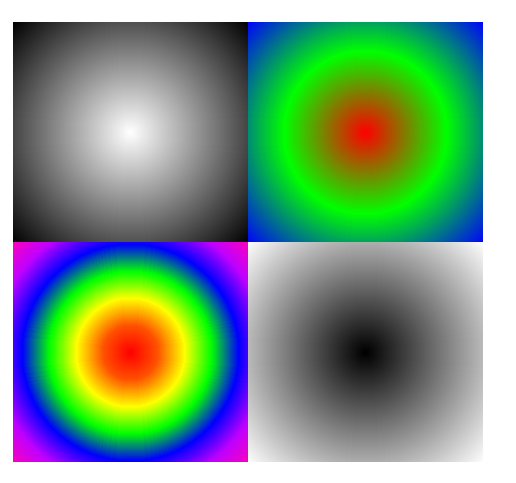Android Code Snippet [B4X] [BitmapCreator] Draw radial gradients

Discussion in 'Code Snippets' started by Erel, May 10, 2018.

1. Currently BitmapCreator supports linear and cubic gradients. This sub creates a radial gradient:Code:
Public Sub FillRadialGradient (bc As BitmapCreator, GradColors() As Int, Rect As B4XRect)

Dim RGBColor(GradColors.Length) As ARGBColor

For i = 0 To GradColors.Length - 1

Next

Dim MaxDistance As Int = Ceil(Sqrt(Power(Rect.Width / 22) + Power(Rect.Height / 22)))

Dim DistanceToColor(MaxDistance) As ARGBColor

Dim CurrentColorIndex As Int

Dim LengthOfEachColor As Int = Ceil(MaxDistance / (GradColors.Length - 1))

Dim c As Int

For i = 0 To MaxDistance - 1

Dim l As Float = CurrentColorIndex / LengthOfEachColor
DistanceToColor(i).a = RGBColor(c).a + l * (RGBColor(c +
1).a - RGBColor(c).a)
DistanceToColor(i).r = RGBColor(c).r + l * (RGBColor(c +
1).r - RGBColor(c).r)
DistanceToColor(i).g = RGBColor(c).g + l * (RGBColor(c +
1).g - RGBColor(c).g)
DistanceToColor(i).b = RGBColor(c).b + l * (RGBColor(c +
1).b - RGBColor(c).b)
CurrentColorIndex = CurrentColorIndex +
1

If CurrentColorIndex = LengthOfEachColor Then
CurrentColorIndex =
0
c = c +
1

End If

Next

Dim cx As Int = Rect.CenterX

Dim cy As Int = Rect.CenterY

For x = Max(0Rect.Left) To Min(bc.mWidth - 1Rect.Right - 1)

Dim dx As Int = Power(x - cx, 2)

For y = Max(0Rect.Top) To Min(bc.mHeight - 1Rect.Bottom - 1)

Dim distance As Int = Sqrt(dx + Power(y - cy, 2))
bc.SetARGB(x, y, DistanceToColor(distance))

Next

Next
End Sub
Usage example:
Code:
Dim bc As BitmapCreator

Dim scale As Float = 100dip / 100
bc.Initialize(ImageView1.Width / scale, ImageView1.Height / scale)

Dim r As B4XRect
r.Initialize(
00, bc.mWidth / 2, bc.mHeight / 2)
Array As Int(xui.Color_White, xui.Color_Black), r)

r.Initialize(bc.mWidth /
20, bc.mWidth, bc.mHeight / 2)
Array As Int(xui.Color_Red, xui.Color_Green, xui.Color_Blue), r)

r.Initialize(bc.mWidth /
2, bc.mHeight / 2, bc.mWidth, bc.mHeight)
Array As Int(xui.Color_Black, xui.Color_White), r)

r.Initialize(
0, bc.mHeight / 2, bc.mWidth / 2, bc.mHeight)
Array As Int(xui.Color_Red, 0xFFFF5200, xui.Color_Yellow, xui.Color_Green, xui.Color_Blue, _

0xFFBF00FF0xFFFF00BB), r)

ImageView1.SetBitmap(bc.Bitmap)

2. With the update to the new BitmapCreator the 'FillRadialGradient' became an own property of the BitmapCreator. The upper function is not necessary any more.

Code: